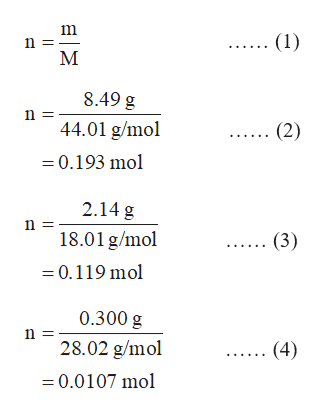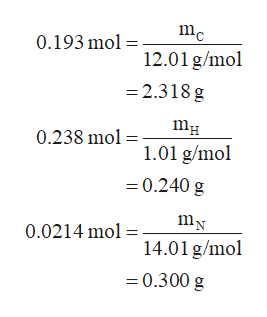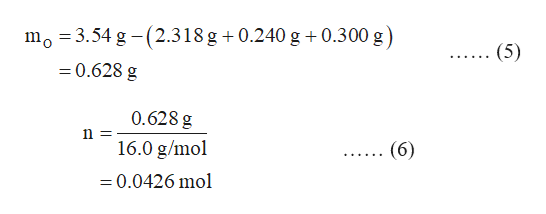# Benzocaine contains only carbon, hydrogen, nitrogen, and oxygen. Complete combustion of 3.54g of pure benzocaine in pure oxygen produced 8.49g of Co2, 2.14g of H2O, and 0.300g of N2. What is the empirical formula for benzocaine?

Question

Benzocaine contains only carbon, hydrogen, nitrogen, and oxygen. Complete combustion of 3.54g of pure benzocaine in pure oxygen produced 8.49g of Co2, 2.14g of H2O, and 0.300g of N2. What is the empirical formula for benzocaine?

check_circleExpert Solution
Step 1

First, calculate the mass of C, H and N atoms present in benzocaine from the mass of CO2, H2O and N2 as follows:

The number of moles of a substance is given by the equation (1) in which m is the given mass of substance and M is the molar mass.

The number of moles of carbon dioxide is calculated in the equation (2) by substituting the its mass and molar mass in equation (1).

As each carbon dioxide molecule contains one carbon atom which comes from the combustion of benzocaine molecule, therefore, the number of moles of carbon atoms in benzocaine is equal to the moles of carbon dioxide that means equal to 0.193 mol.

The number of moles of water is calculated in the equation (3) by substituting the its mass and molar mass in equation (1).

As each water molecule contains two hydrogen atom which comes from the combustion of benzocaine molecule, therefore, the number of moles of hydrogen atoms in benzocaine is equal to the double of the moles of water that means equal to 2 X 0.119 mol = 0.238 mol.

The number of moles of N2 is calculated in the equation (4) by substituting the its mass and molar mass in equation (1).

As each N2 molecule contains two nitrogen atom which comes from the combustion of benzocaine molecule, therefore, the number of moles of nitrogen atoms in benzocaine is equal to the double of the moles of N2 that means equal to 2 X 0.0107 mol = 0.0214 mol.help_outlineImage Transcriptionclose(1) M 8.49 g n 44.01 g/mo (2) 0.193 mol 2.14 g n 18.01 g/mol (3) 0.119 mol 0.300 g n 28.02 g/mol (4) 0.0107 mo | fullscreen
Step 2

The mass of C, H and N atoms present in the benzocaine sample is calculated as follows:help_outlineImage Transcriptionclosemc 0.193 mo 12.01 g/mol 2.318 g 0.238 mol 1.01 g/mol 0.240 g mN 0.0214 mol14.01 g/mol -0.300 g fullscreen
Step 3

The mass of oxygen atoms present in the sample of benzocaine is calculated in the equation (5) by subtracting the mass of C, H and N atoms from the mass of pure benzocaine...help_outlineImage Transcriptionclosemo 3.54 g -(2.318 g 0.240 g 0.300 g) (5) 0.628 g 0.628 g n = 16.0 g/mol (6) - 0.0426 mol fullscreen

### Want to see the full answer?

See Solution

#### Want to see this answer and more?

Solutions are written by subject experts who are available 24/7. Questions are typically answered within 1 hour*

See Solution
*Response times may vary by subject and question
Tagged in

### Chemistry